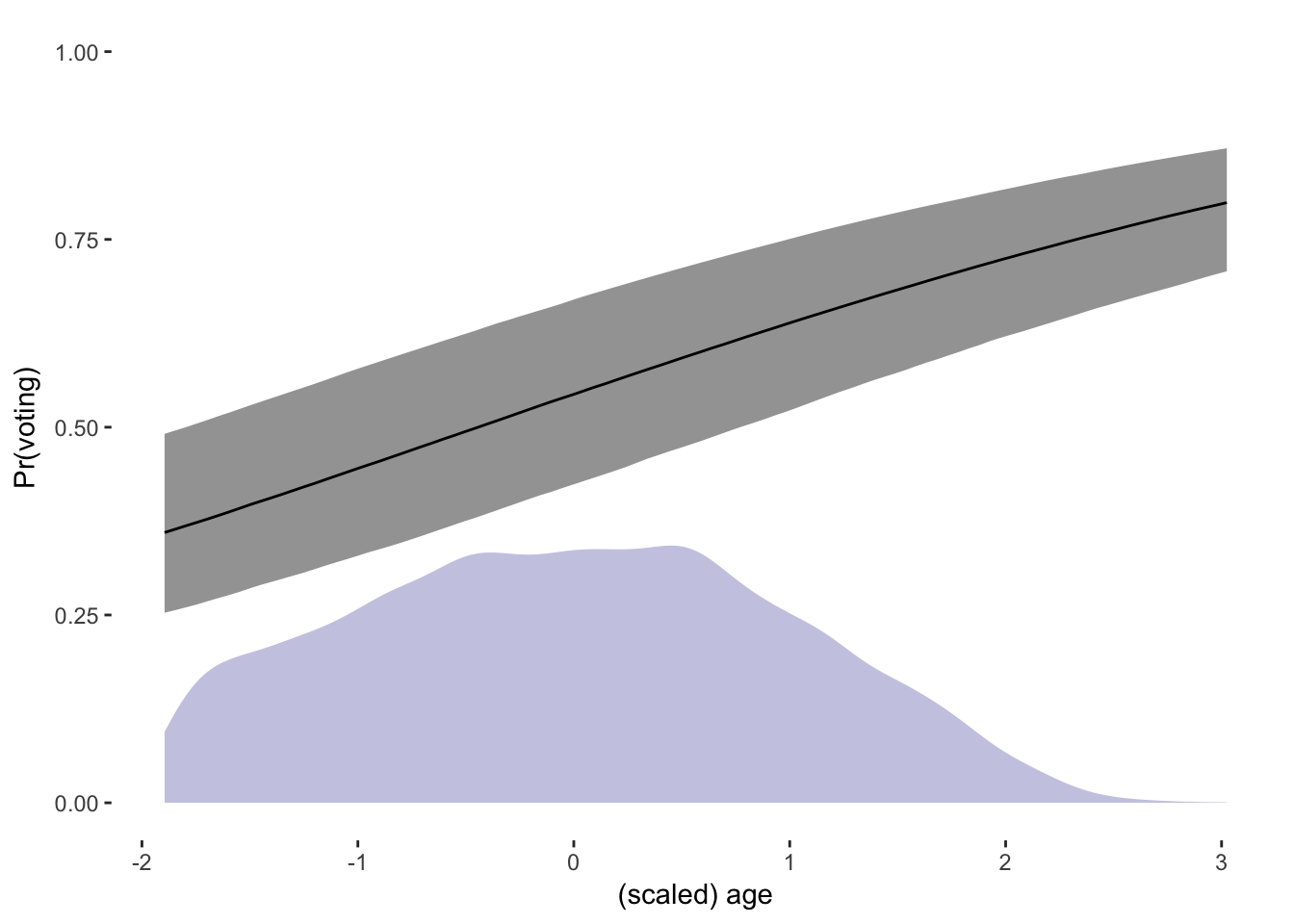Using data on voting behavior in European elections from http://www.jkarreth.net/files/full_cses3.dta, estimate a Bayesian logistic regression that predicts whether someone voted or not in 2007 as a function of their age, gender, education, and income. Present point estimates and measures of uncertainty for coefficient posterior distributions in a table. Calculate the predicted probability of voting as a function of age, and present in graphical form.

If you don’t want to write your own Stan model, there’s one available on my GitHub. Johannes Karreth has helpfully written a function that automatically performs predicted probability calcuations on the output of a Bayesian model. Combining the summary() function with kable() can produce a serviceable regression table, but I’ve written a function which makes much nicer looking tables with minimal effort.

library(rstan) # Bayesian inference
rstan_options(auto_write = TRUE) # avoid recompiling model
options(mc.cores = parallel::detectCores()) # parallelizing multiple chains
library(xtable) # table output

# read in data from Johannes Karreth's website and subset to 2007
elections <- rio::import('http://www.jkarreth.net/files/full_cses3.dta')
elections <- elections[grepl('2007', elections$election), ] # drop NAs in right and left side variables elections <- na.omit(elections[, c("voted", "age", "female", "education", "income")]) # response variable Y <- elections$voted

# predictors
X <- model.matrix(voted ~ scale(age) + female + as.factor(education)
+ as.factor(income), data = elections)

# create named list of data to pass to Stan
stan_data <- list(Y = Y, X = X, N = nrow(X), K = ncol(X))

destfile = 'logit.stan')

# fit model
vote_fit <- stan(file = 'logit.stan', data = stan_data, pars = 'beta',
iter = 1000, chains = 4)

# rename betas
names(vote_fit)[1:14] <- gsub('as.factor', '', colnames(X))

# source MCMC regression table function
source('https://raw.githubusercontent.com/jayrobwilliams/RWmisc/master/mcmcreg.R')

# create regression table
mcmcreg(vote_fit, pars = 'beta', format = 'html', caption = 'Bayesian logistic regression.
Point estimates are posterior means.')
Bayesian logistic regression. Point estimates are posterior means.
Model 1
(Intercept) 0.47
[-0.02; 0.98]
scale(age) 0.40*
[0.35; 0.45]
female -0.28*
[-0.37; -0.19]
(education)2 0.57
[-0.03; 1.16]
(education)3 0.97*
[0.45; 1.47]
(education)4 0.52
[-0.01; 1.02]
(education)5 0.70*
[0.16; 1.19]
(education)6 0.53*
[0.01; 1.02]
(education)7 1.27*
[0.71; 1.81]
(education)8 1.33*
[0.79; 1.86]
(income)2 0.40*
[0.28; 0.52]
(income)3 0.66*
[0.52; 0.79]
(income)4 0.91*
[0.77; 1.06]
(income)5 1.10*
[0.94; 1.25]
* 0 outside 95% credible interval
# source predicted probabilities function
source('https://raw.githubusercontent.com/jkarreth/JKmisc/master/MCMC_simcase_probs.R')

# extract post-warmup beta draws from all four chains
vote_betas <- extract(vote_fit, pars = 'beta')\$beta

# calculate predicted probabilities based on median of observed variables
vote_pp <- MCMC_simcase_probs(model_matrix = X, mcmc_out = vote_betas, x_col = 2,
x_range_vec = seq(min(X[, 2]), max(X[, 2]), length.out = 100),
lower = .025, upper = .975)

# source clean ggplot theme
source('https://raw.githubusercontent.com/jayrobwilliams/RWmisc/master/theme_rw.R')

# plot predicted probabilities, with density plot of observed values
ggplot(data = vote_pp, aes(x = predictor, y = median_pp, ymin = lower_pp, ymax = upper_pp)) +
geom_ribbon(alpha = .5) +
geom_line() +
geom_density(data = elections, aes(x = as.numeric(scale(age))), inherit.aes = F, color = NA,
fill = 'darkblue', alpha = .25) +
coord_cartesian(ylim = c(0,1)) +
labs(x = '(scaled) age', y = 'Pr(voting)') +
theme_rw()